# Thread: Differentiation Questions

1. ## Differentiation Questions

Hi
Can someone tell me if my answers are correct for the following questions:

1) Find the slope of the graph$\displaystyle y=2x^3 + 4\sqrt{x}$ at x = 2

$\displaystyle \frac{dy}{dx} = 6x^2 + 2x^{\frac{-1}{2}}$

when x = 2

$\displaystyle \frac{dy}{dx} = 24 + \frac{1}{2\sqrt{2}}$

so the slope at x=2 is 24.3536

2)Evaluate the derivative: $\displaystyle \frac{d}{dx}[\frac{x^2-5x+1}{x^2+2x-1}]$

$\displaystyle = \frac{2x-5}{2x+2}$

3) Find $\displaystyle \frac{dx}{dt}$ when $\displaystyle x = (5-3t)^{4t}$

$\displaystyle \frac{dx}{dt} = 4t(5-3t)^{4t}$

$\displaystyle \frac{dx}{dt} = -12t(5-3t)^{4t-1}$

P.S

2. in the first example it is
$\displaystyle \frac{dy}{dx} = 6x^2 + \sqrt{2}$

you put the 2 in the denominator instead of numerator and thats why you got the wrong result...

2)
this is actually much more complicated than that, look up quotient rule you will find it usefull.

the way you solve this is

$\displaystyle (\frac{d}{dx}) \frac{f(x)}{g(x)} = \frac{\frac{df}{dx}g(x)-f(x)\frac{dg}{dx}}{g(x)^2}$

the result is
$\displaystyle \frac{7x^2-4x+3}{(x^2+2x-1)^2}= \frac{dy}{dx}$

3) in the third question i think you need to do

$\displaystyle \frac{dy}{dx}= -12(5-3t)^{4(t-1)}$

but im not 100% sure so you might want a second opinion on that

3. see attachment4. One way to tackle no. 3 is the chain rule for two inner functions...

$\displaystyle \frac{d}{dx}\ f(u(x), v(x)) = \frac{\partial f}{\partial u} \frac{du}{dx} + \frac{\partial f}{\partial v} \frac{dv}{dx}$

See http://www.wolframalpha.com/input/?i...%29%5E%284t%29 (and click on 'show steps') for the orthodox working with that rule.

Just in case a picture helps...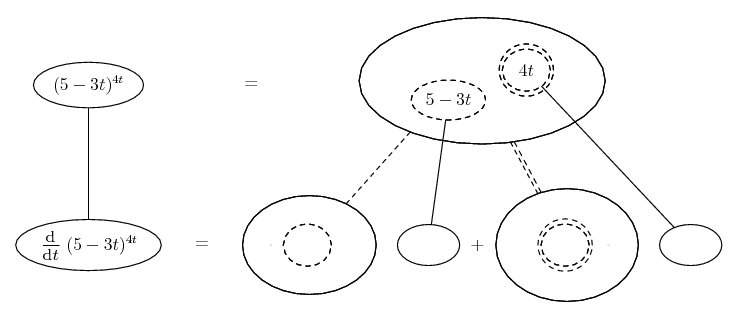... where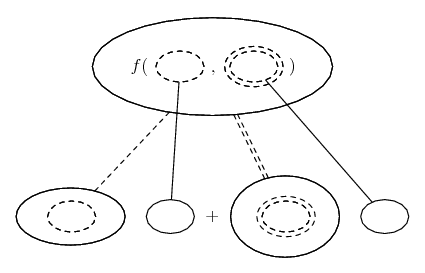... is the above rule, i.e. a double version of...... the ordinary chain rule. Straight continuous lines differentiate downwards (integrate up) with respect to t, and the straight dashed lines similarly but with respect to their corresponding dashed balloon expression (the inner function of the composite which is subject to the chain rule).

Spoiler: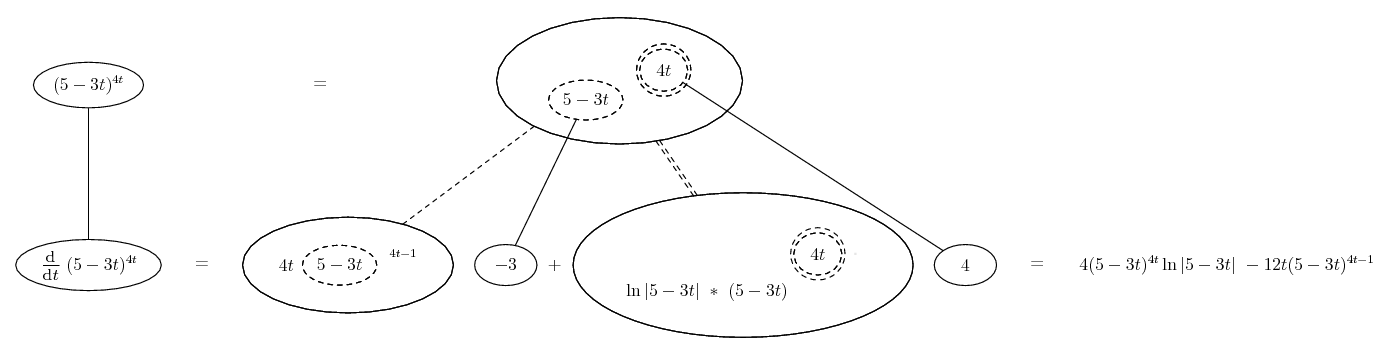Another way is to take natural logs of both sides...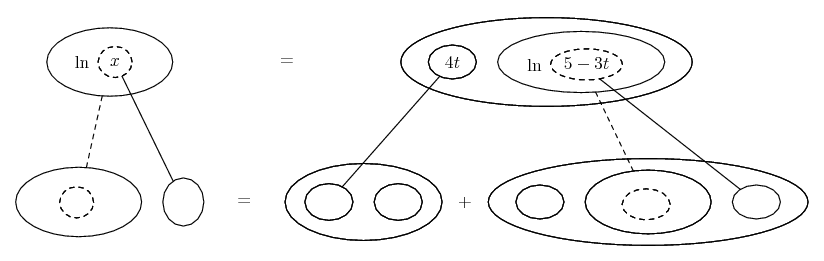Spoiler: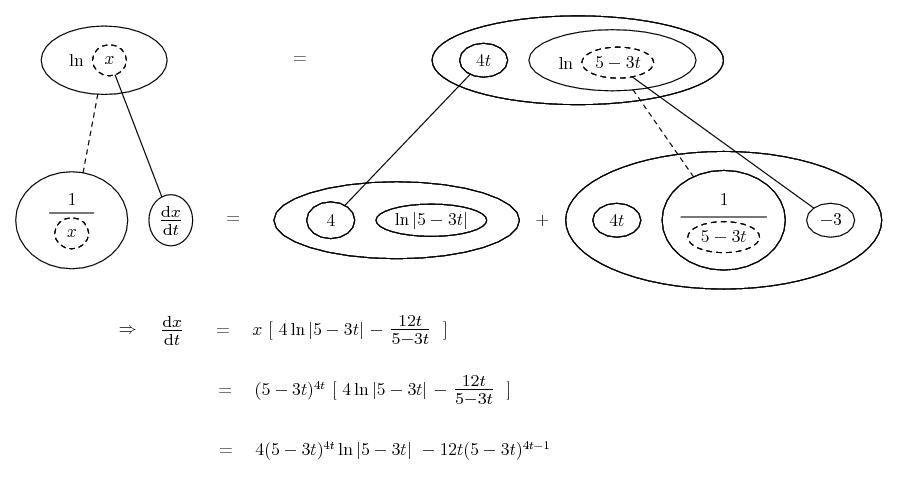... with...... the product rule

(more in a tic).
_________________________________________
Don't integrate - balloontegrate!

Balloon Calculus; standard integrals, derivatives and methods

Balloon Calculus Drawing with LaTeX and Asymptote!

#### Search Tags

differentiation, questions